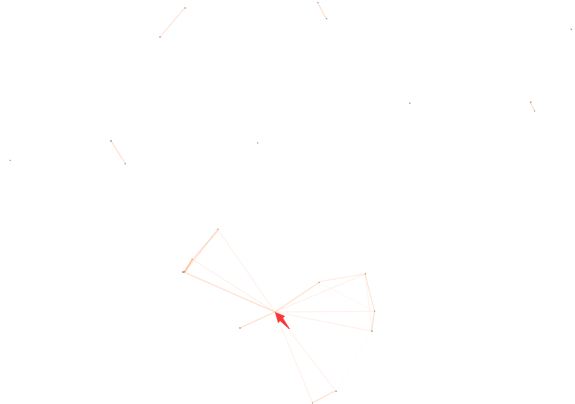## html线条动态背景(线条随鼠标变动)

1533 0 0js代码

``<script type="text/javascript" src="http://apps.bdimg.com/libs/jquery/2.1.4/jquery.min.js"></script>``
``````! function () {
function n(n, e, t) {
return n.getAttribute(e) || t
}
function e(n) {
return document.getElementsByTagName(n)
}
function t() {
var t = e("script"),
o = t.length,
i = t[o - 1];
return {
l: o,
z: n(i, "zIndex", -1),
o: n(i, "opacity", .5),
c: n(i, "color", "255,97,6"),
n: n(i, "count", 99)
}
}
function o() {
a = m.width = window.innerWidth || document.documentElement.clientWidth || document.body.clientWidth,
c = m.height = window.innerHeight || document.documentElement.clientHeight || document.body.clientHeight
}
function i() {
r.clearRect(0, 0, a, c);
var n, e, t, o, m, l;
s.forEach(function (i, x) {
for (i.x += i.xa, i.y += i.ya, i.xa *= i.x > a || i.x < 0 ? -1 : 1, i.ya *= i.y > c || i.y < 0 ? -1 : 1, r.fillRect(i.x - .5, i.y - .5, 1, 1), e = x + 1; e < u.length; e++) n = u[e],
null !== n.x && null !== n.y && (o = i.x - n.x, m = i.y - n.y,
l = o * o + m * m, l < n.max && (n === y && l >= n.max / 2 && (i.x -= .03 * o, i.y -= .03 * m),
t = (n.max - l) / n.max, r.beginPath(), r.lineWidth = t / 2, r.strokeStyle = "rgba(" + d.c + "," + (t + .2) + ")", r.moveTo(i.x, i.y), r.lineTo(n.x, n.y), r.stroke()))
}),
x(i)
}
var a, c, u, m = document.createElement("canvas"),
d = t(),
l = "c_n" + d.l,
r = m.getContext("2d"),
x = window.requestAnimationFrame || window.webkitRequestAnimationFrame || window.mozRequestAnimationFrame || window.oRequestAnimationFrame || window.msRequestAnimationFrame ||
function (n) {
window.setTimeout(n, 1e3 / 45)
},
w = Math.random,
y = {
x: null,
y: null,
max: 2e4
};
m.id = l, m.style.cssText = "position:fixed;top:0;left:0;z-index:" + d.z + ";opacity:" + d.o, e("body").appendChild(m), o(), window.onresize = o,
window.onmousemove = function (n) {
n = n || window.event, y.x = n.clientX, y.y = n.clientY
},
window.onmouseout = function () {
y.x = null, y.y = null
};
for (var s = [], f = 0; d.n > f; f++) {
var h = w() * a,
g = w() * c,
v = 2 * w() - 1,
p = 2 * w() - 1;
s.push({
x: h,
y: g,
xa: v,
ya: p,
max: 6e3
})
}
u = s.concat([y]),
setTimeout(function () {
i()
}, 100)
}();``````